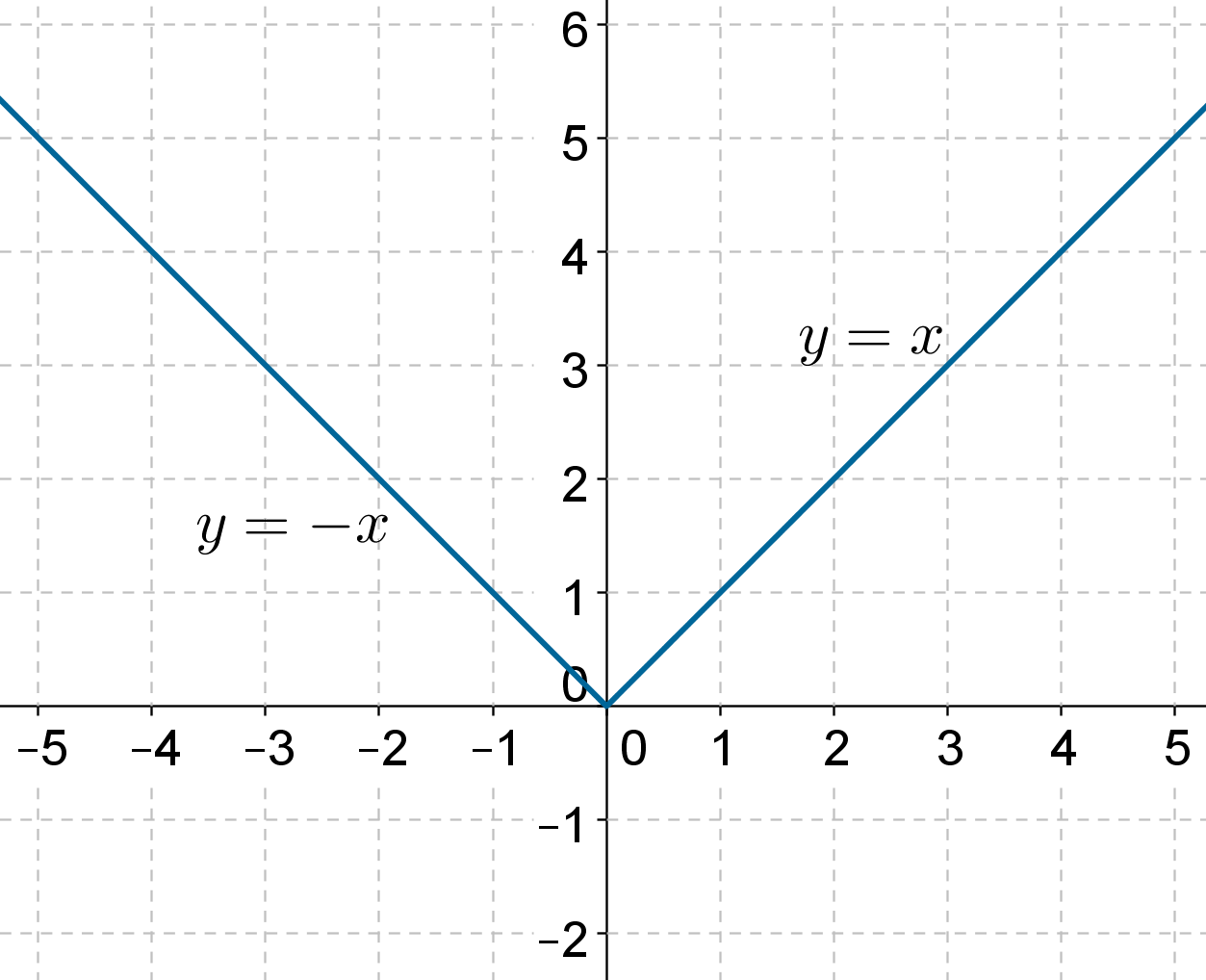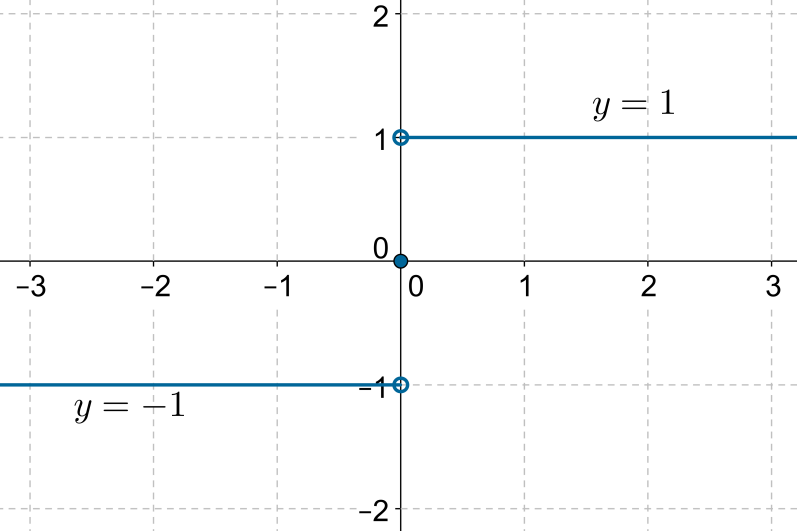# The Modulus Function

Go back to  'Functions'

## What is a modulus function?

The modulus of any number gives us the magnitude of that number. Using the modulus operation, we can define the modulus function as follows:

$f\left( x \right) = \left| x \right|$

Now, if x is non-negative,then the output of f will be x itself. If x is negative,then the output of f will be the magnitude of x, which we can write as $$- x$$ (note that if x is negative,then $$- x$$ will be positive). Thus, we can redefine the modulus function as follows:

$f\left( x \right) = \left\{ \begin{array}{l}\;\;\;x,\,\,\, & if\,x \ge 0\\ - x, & if\,x < 0\end{array} \right.$

The graph of f is plotted below:Note that for positive values of x,the graph is the line $$y = x$$; for negative values of x, the graph is the line $$y = - x$$. This is in line with the piecewise definition of the modulus function.

Since we can apply the modulus operation to any real number, the domain of the modulus function is $$\mathbb{R}$$. The range is clearly the set of all non-negative real numbers, or $$\left( {0,\infty} \right)$$.

Example 1: A function f is defined on  $$\mathbb{R}$$ as follows:

$f\left( x \right) = \left\{\!\!\!\begin{gathered}\frac{{\left| x \right|}}{x},\;\;x\ne 0\\\;\;0,\;\;\;\;\; x = 0\end{gathered} \right.$

Plot the graph of f.

Solution: When x is positive, we have:

$f\left( x \right) = \frac{{\left| x \right|}}{x} =\frac{x}{x} = 1$

When x is negative, we have:

$f\left( x \right) = \frac{{\left| x \right|}}{x} =\frac{{ - x}}{x} = - 1$

Thus, we can redefine f as follows:

$f\left( x \right) = \left\{ \begin{array}{l}\;\;1, & x > 0\\\;\;0, & x = 0\\ - 1, & x < 0\end{array} \right.$

The graph of f is now easy to plotNote the solid dot at $$\left( {0,0} \right)$$, and the two hollow dots at $$\left( {0,1} \right)$$ and $$\left( {0, - 1} \right)$$. This indicates that$$f\left( 0 \right)$$ has the value 0 rather than 1 or $$- 1$$.

This particular function is known as the  signum  function.

Functions
Functions
grade 10 | Questions Set 2
Functions
Functions
grade 10 | Questions Set 1